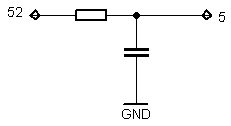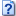# Very simple Arduino capacitance meter using 16 bit timer and analog comparator

As You could see from video it requires only one external resistor. Meter is very simple, but obviously not very accurate or wide range. Theoretically it could measure in 10 nF – 160 µF range.

How it Works?

Circuit formed from resistor and capacitor (RC circuit) has time constant, it shows time needed to discharge capacitor via resistor to ~37% it’s initial voltage. Calculation of time constant is very simple t=R*C . For 1 k resistor and 47µF capacitor it’s 47ms.My capacitance meter fully charges capacitor, when 16 bit timer is started and capacitor is discharging via resistor (1 k). Capacitor is connected to analog voltage comparator(pin 5), as capacitor voltage drops bellow 1.1 V occurs analog comparator interrupt which triggers timer interrupt.  If resistor is constant, discharge time and capacitance dependency is linear, therefore if You know one rated capacity capacitor discharge time, You could easily calculate another capacitor’s capacitance by measuring time.

Because timer uses only one  /64 clock prescaler measurement range is very narrow. To have wider range there is a always way to make  programmable prescaler, which changes if capacitor value is out of range, or discharge capacitor via range of different resistors.

Also keep in mind that resistor’s resistance depends on environment temperature, consequently and on LCD display showed capacitance. To fix this temperature dependency referenced capacitor should be used. In this case every time both capacitors are charged and discharged separately, but via the same resistor. Capacitance proportion is calculated and if referenced capacitor capacitance is know measured capacitor’s value can be calculated just by division or multiplying.

It’s time for program’s code.

Display shows OVF if timer’s counter reached max value, but capacitor’s voltage isn’t dropped to required level. It mean that where isn’t capacitor inserted, o it is out of measurement range.

```ISR(TIMER1_OVF_vect) {
ovf=1;
lcd.clear();
lcd.setCursor(0, 0);
lcd.print("OVF");

}```

Initialization

```void setup() {

TCCR1B=0x00; //stop timer

lcd.begin(16, 2);
lcd.print("electronicsblog");
lcd.setCursor(0, 1);
lcd.print(".net");
delay(1000);

TCCR1A = 0x00; //normal timer mode
TIMSK1=0x21; //Interrupt Mask Register for timer 1

pinMode(discharge_pin, OUTPUT);

}```

TIMSK1=0x21;  It enables Input Capture and Overflow interrupts.

Main routine

```void loop() {

level=1;

ACSR=0x80; //analog comparator is turned off

pinMode(comparator_pin, OUTPUT);
//Capacitor charging starts via 2 pins
digitalWrite(comparator_pin, HIGH);
digitalWrite(discharge_pin,HIGH);

delay(100);

//preparing comparator
pinMode(comparator_pin, INPUT);
digitalWrite(comparator_pin, LOW);

ACSR=0x47; // Analog Comparator Control and Status Register
DIDR1=0x03; //Digital Input Disable Register

TCNT1=0x00; // counter reset to zero;

pinMode(discharge_pin, OUTPUT);
digitalWrite(discharge_pin,LOW); // starting capacitor discharge
TCCR1B = 0xC3; //start timer (clock prescaler /64)

//wait till capacitor discharges to required level,
// or overflow occurs
while (level&&!ovf) {delay(10);}

}```

ACSR=0x80; Analog Comparator Control and Status Register, set bit 7 – ACD: Analog Comparator Disable.

ACSR=0x47; (bit 6) fixed bandgap reference voltage(1.1 V) replaces the positive input to the Analog  Comparator. (bit 2) Input capture function in Timer/Counter1 to be triggered
by the Analog Comparator is enabled. (bit 1,0) Comparator Interrupt on Falling Output Edge.

DIDR1=0x03; digital input buffer on the AIN1/0 (comparator) pin is disabled.

Timer 1 Capture Event interrupt

```ISR(TIMER1_CAPT_vect) {

TCCR1B=0x00; //stop timer
counter=ICR1; //saving counter value to variable
level=0; // capacitor's voltage reached required level
ACSR=0x80; //analog comparator is turned off

if (counter>3) { // to avoid false result with no capacitor
if (!ovf) {lcd.clear();}
lcd.setCursor(0, 1);
// because with 200nF capacitor counter stopped by 82.
cap=(double)counter/82.0*200.0;

// showing result in nF or µF automatically
if (cap<1000) {lcd.print(cap); lcd.print(" nanoF");}
else {lcd.print(cap/1000.0); lcd.print(" microF");}
}

ovf=0;

}```Very simple Arduino capacitance meter source code (1.9 KiB, 3,800 hits)